# OR-Notes

## J E Beasley

OR-Notes are a series of introductory notes on topics that fall under the broad heading of the field of operations research (OR). They were originally used by me in an introductory OR course I give at Imperial College. They are now available for use by any students and teachers interested in OR subject to the following conditions.

A full list of the topics available in OR-Notes can be found here.

#### Network analysis - extensions (brief overview)

There are several important extensions to the basic network analysis technique and these relate to:

We shall deal with each of these in turn. More details can be found in the links for each of these extensions given above.

#### Uncertain activity completion times

One important extension to the basic network analysis technique relates to uncertain activity completion times. In this extension to the basic method we give, for each activity, not a single completion time but three times:

• optimistic time (t1) - the completion time if all goes well
• most likely (modal) time (t2) - the completion time we would expect under normal conditions
• pessimistic time (t3) - the completion time if things go badly.

This use of three time estimates is the PERT technique. Note here that these three time estimates do not imply that, when the activity is performed, its completion time will be one of three choices - optimistic, most likely or pessimistic. Instead these three numbers are used to produce an underlying probability distribution of completion times such that all times are possible (with an associated probability).

These three times are combined into a single number, the expected activity completion time, given by (t1 + 4t2 + t3)/6. Once we have a single number then we can do exactly the same calculation as before with this single number being used as the activity completion time in order to find the project completion time and the critical activities.

Note here that this weighting of optimistic:most likely:pessimistic of 1/6:4/6:1/6 is essentially fixed and cannot be altered (as the underlying theory depends on these weights).

In addition, through the use of the beta and normal probability distributions, we can get an idea of how this project completion time might vary (remember we no longer know the individual activity completion times with certainty).

Essentially we can find answers to questions like:

• What is the probability that the project will take longer than...?
• What is the probability that the project will be finished by...?

To illustrate this consider the package input shown below where, for the activity on node network we had before, (also reproduced below) we now have an optimistic time equal to one-half the most likely time and a pessimistic time equal to twice the most likely time.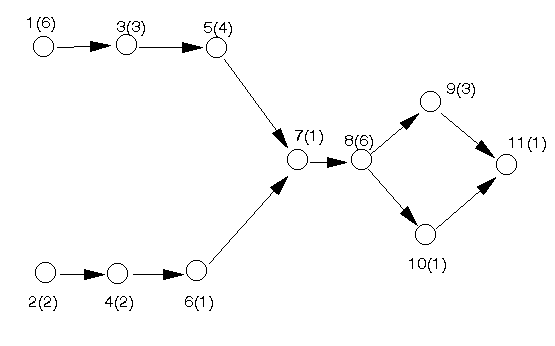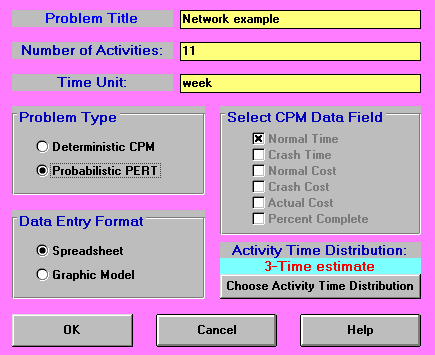In the input shown above we have specified a 3-time estimate for the activity time distribution. The package allows us to alter this (to a considerable extent), but we must be sure that our implicit assumption as to which activity time distribution applies is justified.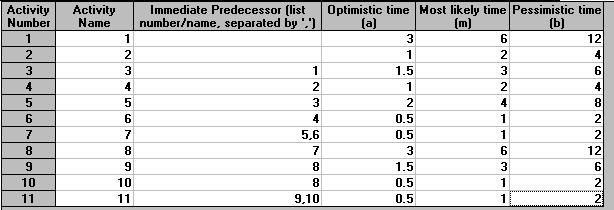The output from the package for this example is shown below. Note here that one of the columns in that figure corresponds to the expected (mean) activity completion time of (t1 + 4t2 + t3)/6. All of the earliest/latest start/finish time calculations are performed with respect to this expected (mean) time. You can see from below that we expect to finish this project in 26 weeks.

`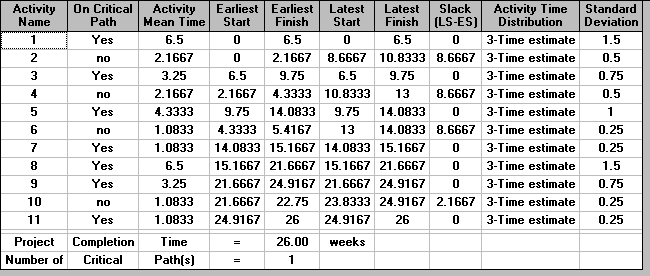`

In the above output the final column is labelled Standard Deviation. As we have a statistical distribution for the completion time of each activity (as represented by the optimistic, most likely and pessimistic times) we also have an underlying statistical variation in the completion time - as represented by the standard deviation. This standard deviation is calculated as (t3 - t1)/6. The critical path is shown below.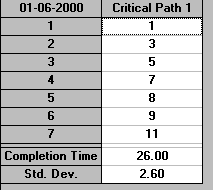#### Probability analysis

Below we show the probability analysis obtainable from the package where, for any project completion time, we have an estimate of the probability of finishing the project within that time. In the example below we have chosen to estimate the probability of completing the project within 27 weeks.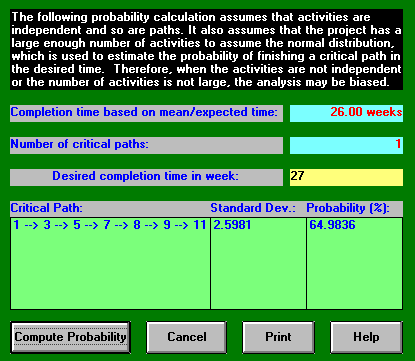It can be seen that although the expected completion time is 26 weeks the probability of completing within any time up to and including 27 weeks is 64.9836%. We can also carry out this probability calculation for a number of other completion times, as below for 25 weeks and 26 weeks.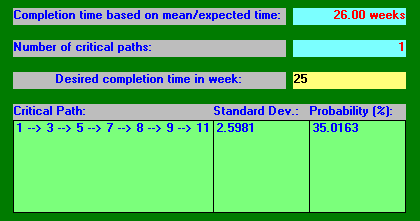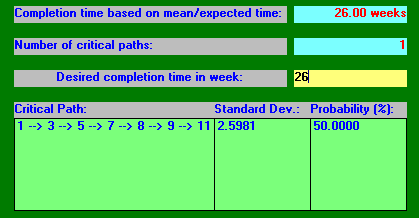Note especially here how the probability of completing within the expected completion time of 26 weeks is only 50%.

Using this probability analysis it is possible to construct the graph shown below where, for a range of possible project completion times, we have plotted the probability that the project has been completed by that time.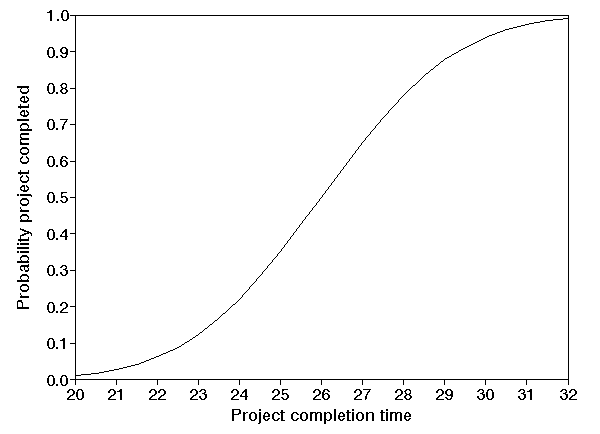Note here that the curve shown above is not fixed - we may be able to change its shape by refining the time estimates (optimistic, most likely, pessimistic) we used which led to the curve, i.e. to reduce the uncertainty associated with each activity, and hence reduce the uncertainty associated with the completion of the entire project.

In this extension to the basic method we assume that, for each activity, the completion time can be reduced (within limits) by spending more money on the activity. Essentially each activity now has more than one possible completion time (depending upon how much money we are willing to spend on it).

This use of cost information is the CPM technique.

A common assumption is to say that for each activity the completion time can lie in a range with a linear relationship holding between cost and activity completion time within this range (as illustrated below).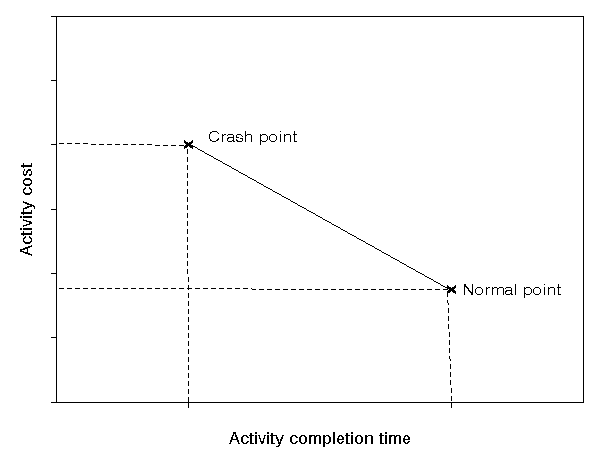Reducing an activity completion time is known as "crashing" the activity and to illustrate this concept consider the activity on node network we had before, for which the network diagram is reproduced below.Introducing costs into this problem we have the package input shown below. For activity 1, for example, the normal cost is 100 associated with a normal time of 6 weeks, and the crash cost is 240 associated with a crash time of 4 weeks.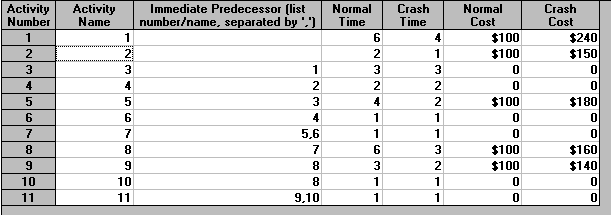The output from the package for this example is shown below.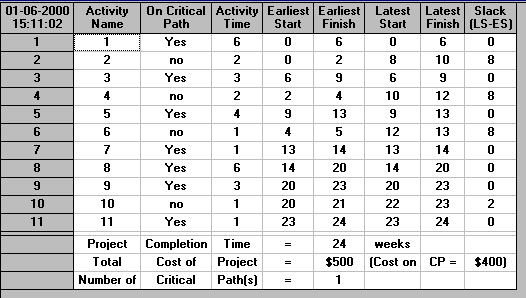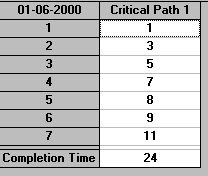The above calculation is based upon the normal times for each activity. If we were to adopt the crash times for each and every activity we would have: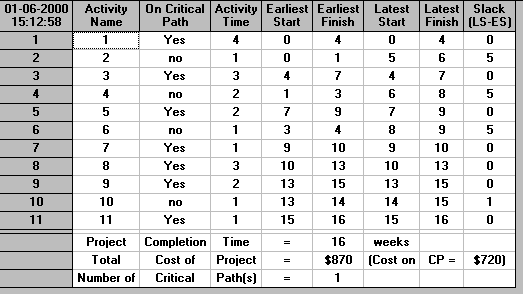indicating that the project can take anywhere between 24 and 16 weeks depending upon the activity completion time (which can vary between the normal time and the crash time).

#### Package crashing

The package calculates the optimal (minimum cost) way to crash the project using linear programming. As discussed elsewhere we can formulate a network problem using linear programming. This formulation can be easily modified to deal with the problem of crashing a project.

The advantage of using linear programming to crash a project is that we can automatically guarantee that, for any particular project completion time, we have achieved that time by crashing in a minimum cost fashion.

The package output giving the cost associated with crashing the project from its normal completion time of 24 weeks to 19 weeks (for example) is given below.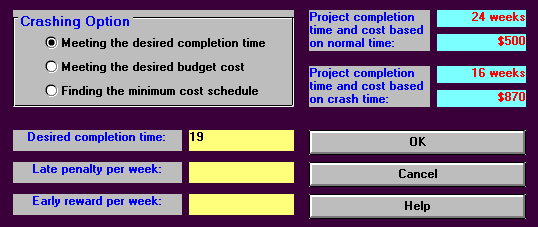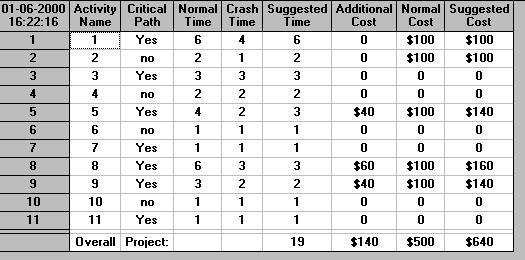It can be seen that the minimum cost way to achieve an overall project completion time of 19 weeks is by crashing activity 5 by one week, activity 8 by three weeks and activity 9 by one week.

The output below shows the minimum cost way of achieving the lowest possible overall project completion time of 16 weeks. It can be seen that this can be done for a cost of 820. This contrasts with the cost of 870 associated with using all activities at their crash times. The difference arises because it is not necessary to crash all activities to their maximum extent to achieve an overall project completion time of 16 (in this case activity 2 does not need to be crashed).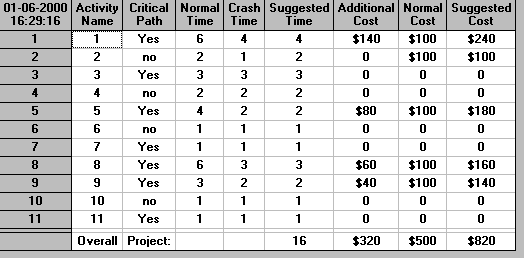By varying the number of weeks by which we crash the project we can construct the graph shown below. In that graph we have plotted, for each possible project completion time, the minimum cost associated with achieving that completion time.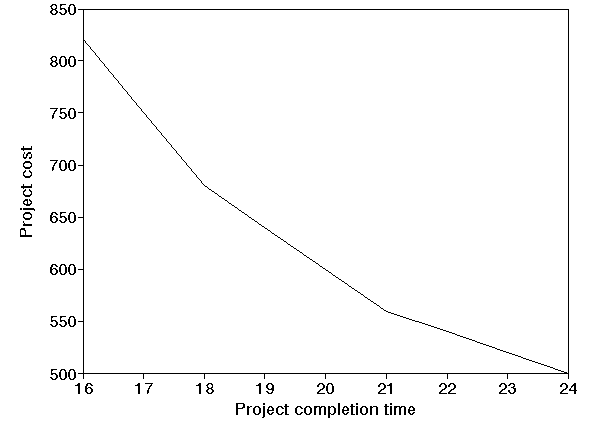Note here that this graph contains three distinct straight line segments (16 to 18, 18 to 21, 21 to 24). In general it is always true that the cost/time tradeoff graph contains a number of distinct straight line segments (i.e. mathematically we would say that it is piecewise linear). This arises because of the linear relationship that was assumed to hold between cost and completion time for each activity.

Note there that the package merely provides information, in this case the cost of the project for all possible completion times between 16 and 24 weeks. It does not tell you which completion time you should choose (as you can have any completion time between 16 and 24 weeks provided you are prepared to pay for it!). What the package does is enable you, as the project manager, to make an informed choice about the completion time to have.

#### Resource restrictions

Typically, in real-world network analysis, each activity has associated with it some resources (such as men, machinery, materials, etc). We mentioned before that, in calculating the minimum overall project completion time, we took no account of any resource restrictions. To illustrate how network analysis can be extended to deal with resource restrictions consider the activity on node network we had before in the case of certainty with respect to activity completion times, for which the network diagram is reproduced below.The Gantt chart below (taken from the package output) illustrates when each activity can take place. To meet the minimum project completion time of 24 weeks for the above project critical activities must take place at fixed points in time. For non-critical activities however we have flexibility (within limits) as to precisely when these activities take place.

To remind you of the interpretation of the Gantt chart below the package displays both the earliest and latest times for each activity and the critical path for the project (using suitable colours). Each activity has two horizontal bars. The first bar represents when it will take place if it is started at its earliest start time. The second bar represents when it will take place if it is started at its latest start time. Obviously for critical activities the two bars are identical.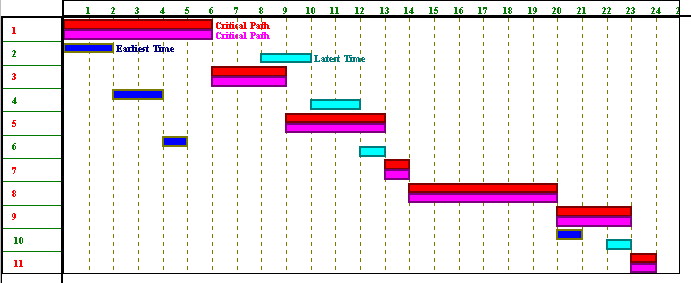Hence for this project the critical activities are 1,3,5,7,8,9 and 11 and the non-critical activities are 2,4,6 and 10.

We consider two different types of resource restricted problem:

• resource smoothing (also known as resource levelling) problems
• resource constrained (also known as resource allocation) problems

Note here that dealing effectively with resource restrictions is hard (both computationally and theoretically). Resource constrained problems are very hard to solve and resource smoothing problems, although easier, are also hard to solve.

The word resource here is used to denote any item (e.g. men, machinery, materials, cash) needed to ensure activities take place. Resources can essentially be classified as:

• renewable per time period (e.g. we have a certain number of men that are available each and every time period); or
• non-renewable over the lifetime of the project (e.g. we might have a certain budget for the project and each sum of money that is spent eats into this budget).

#### Resource smoothing

Suppose now that we have just one resource (men) associated with each activity and that the number of men required are:

• 2 men for activity 1
• 1 man all the other activities (activities 2 to 11 inclusive)

If you click here you will be able to download an Excel 97 spreadsheet called res1.xls with which you can explore using resources in the network above. The examples given below are produced using this spreadsheet.

The spreadsheet is shown (in part) below. Column B in that spreadsheet gives the resource usage for each activity, column C a suggested start time (if we wish to impose our own suggested start time for each activity). Columns D and E show the start and finish time for each activity. The values for the cells in these columns are set using the underlying precedence relationships in the network seen above. Cell D13 shows the end time (when all activities have been completed, i.e. the project completion time). There are other cells in the spreadsheet that contain values but these are either concerned with internal calculations in order to calculate a resource profile or are for features that we have yet to discuss.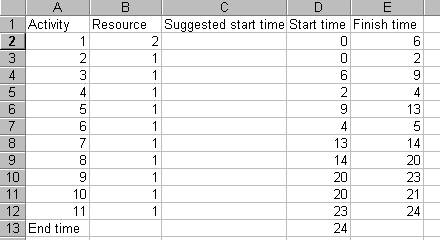Suppose now that we decide:

• we wish to meet the minimum overall project completion time of 24 weeks (hence implying that the times at which critical activities occur are fixed)
• we wish to start all non-critical activities at their earliest possible start times

then in the light of these decisions what does the plot (profile) of resource usage (number of men used) against time look like?

Using our spreadsheet we can see that the plot of resource usage against time is as below.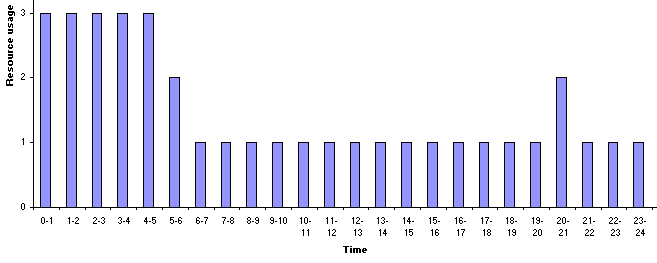The peak of the resource profile is associated with the start of the project, when activity 1 requires 2 men and the other activities, which are being performed simultaneously with activity 1, require one man.

A key question is:

What resource usage profile would you have most liked to have seen here?

Clearly what we would have most liked to have seen here is a constant profile of resource against time, i.e. a constant usage of resource over time. This is because variations from a constant (straight line) profile most likely cost us extra money - either in terms of hiring extra resource to cover peaks in the resource profile, or in terms of unutilised resources when we have troughs in the resource profile. Whilst it may not be possible to achieve an ideal resource profile we should keep this ideal in mind.

Now another key question is:

Is the resource profile that we see fixed or can it be altered?

The simple answer is that the resource profile is not fixed. It can be altered. Simply put changing the start time for an activity changes the resource profile. This is illustrated below where we have changed the start time for activity 2 to time 2. It can be seen that the project still completes in 24 weeks, but the resource profile is different.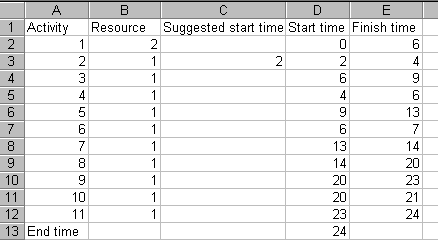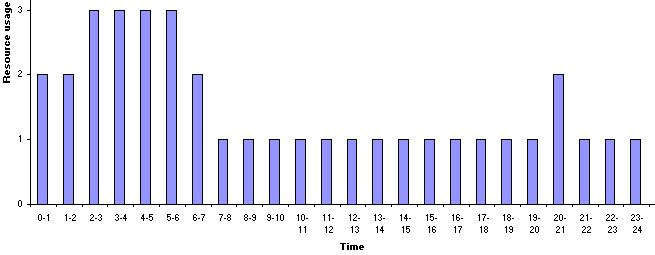So is our current usage of resource ideal? Plainly not, but what flexibility do we have? If we still wish to complete in 24 weeks we can do nothing with regard to the critical activities.

However we have some choice for the non-critical activities. Recall that such activities have an associated float (slack) time. We could artificially delay starting some of these activities. If we do so the resource profile will change, maybe for the better. Indeed this is what we did implicitly above. There, in delaying the start of activity 2 until time 2, we still completed the project on time, but had a different resource profile. In fact for this particular example it is easy to see that delaying starting activity 2 until time 6 leads to a better resource profile, and is still feasible in terms of the 24 weeks completion time.

Using our spreadsheet to delay starting activity 2 until time 6, when activity 1 will have finished we have: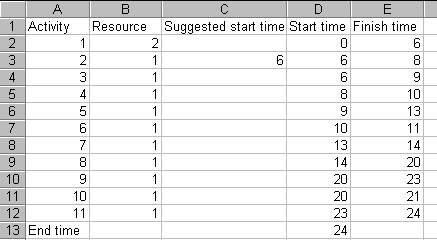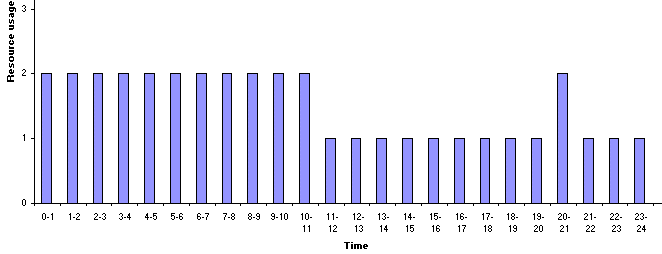Here we clearly have a resource profile more in line with our ideal of a constant resource usage.

It is important to note however that artificially delaying the start of non-critical activities in order to improve a resource profile is not free. Rather it costs us. Simply put time lost by delaying the start of an activity cannot later be regained (if things do not turn out as planned) given the desired fixed completion time for the overall project.

This has illustrated the resource smoothing or resource levelling problem, which can be stated as:

Given a fixed overall project completion time (which we know is feasible with respect to the resource constraints) "smooth" the usage of resources over the timescale of the project (so that, for example, we do not get large changes between one week and the next in the number of men we need).

This smoothing process makes creative use of float to artificially delay activities in order to smooth resource usage.

There are two disadvantages to smoothing:

• if we have multiple resources then we may well find that smoothing one resource makes another resource less smooth, hence we have to make tradeoffs between resource profiles
• time lost cannot be regained, once we have delayed an activity to smooth a resource profile then, if things go wrong later (e.g. some activities take longer than we planned) we cannot regain the time we have lost

#### Resource constrained problems

In some circumstances however we have an absolute constraint upon the maximum level of resource that can be used in any time period. To illustrate this if you click here you will be able to download an Excel 97 spreadsheet called res3.xls with which you can explore resource constrained problems in the network we are considering. The examples given below are produced using this spreadsheet.

To remind you of it the network for the project we are considering is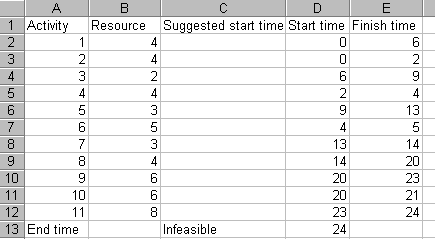The resource profile for the start times shown above is as below.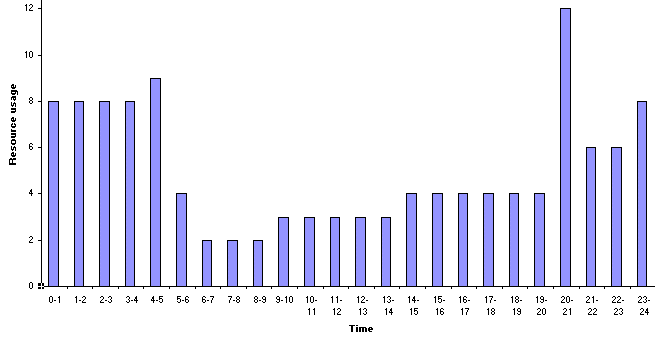Now suppose that we wish to impose an absolute limit of 8 on the usage of this resource in any time period. The current resource profile violates this constraint and this is indicated by cell C13 in the spreadsheet saying "Infeasible".

Whilst we know that from the time point of view the minimum project completion time is 24 weeks a key question is: can we still achieve the same minimum project completion time when a resource constraint is added?

The answer to this question is that sometimes it is impossible to achieve the same completion time. Reflect for a moment that the procedure we have used to calculate the minimum completion time for any project is calculated with no regard to resources. Once we introduce resources and a resource limit then it may (obviously) not be possible to still achieve this completion time.

For the problem we are considering here, for example, activities 9 and 10 are performed simultaneously in achieving the minimum overall project completion time of 24 weeks (e.g. consider the network diagram shown above). Activity 9 requires 6 resource units, activity 10 requires 6 resource units. It is clear therefore that, in the presence of a resource constraint of 8 units these two activities cannot be performed simultaneously. Hence for this network we immediately know that the original completion time of 24 weeks is no longer feasible.

The question therefore arises as to: what is the minimum overall project completion time for the project when resource constraints are taken into account?

This has illustrated the resource constrained or resource allocation problem, which can be stated as:

Given the activities, their associated resource usage, precedence relationships and resource restrictions (typically expressed as the amount of each resource available per time period) find the minimum overall project completion time which satisfies both the precedence relationships and the resource restrictions.

Note here that resource constrained problems are much harder (both theoretically and computationally) to solve than resource smoothing problems. This will become apparent computationally below if you use the spreadsheet to attempt to solve a resource constrained problem.

#### Estimating the minimum project completion time

In order to gain some insight into how we might go about estimating the minimum project completion time when we have a resource constraint of 8 we can start from the resource unconstrained solution. This is shown below together with the resource profile and Gantt chart.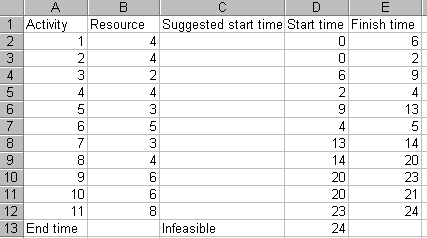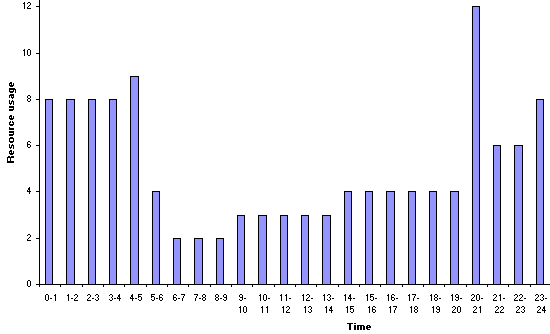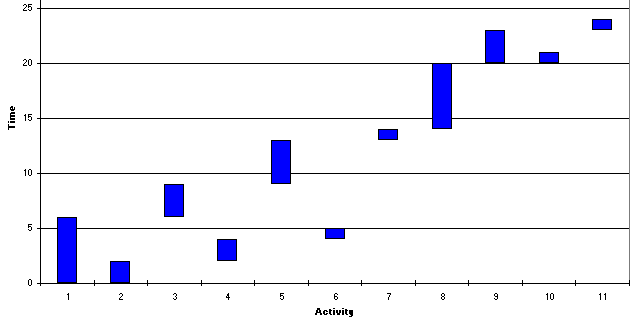Examining the resource profile the first point at which we exceed the resource constraint of 8 is at time 4-5. Examining the Gantt chart this is associated with activity 6 (requiring 5 resource units) being performed simultaneously with activity 1 (which requires 4 resource units). Plainly one (or other) of these activities needs to be delayed. Here, as at time 4-5 we have already started activity 1, we choose to delay activity 6 until activity 1 has finished. Hence we have a suggested start time for activity 6 of 6. Entering this into the spreadsheet we get: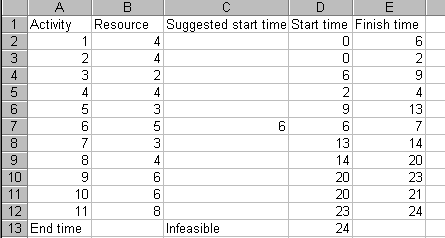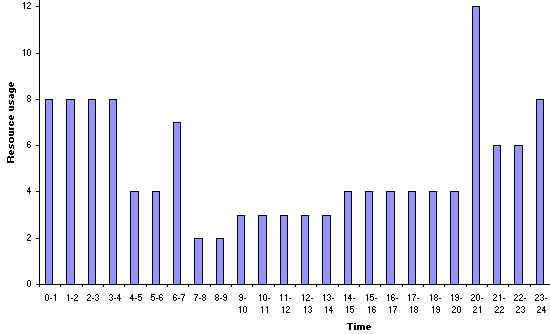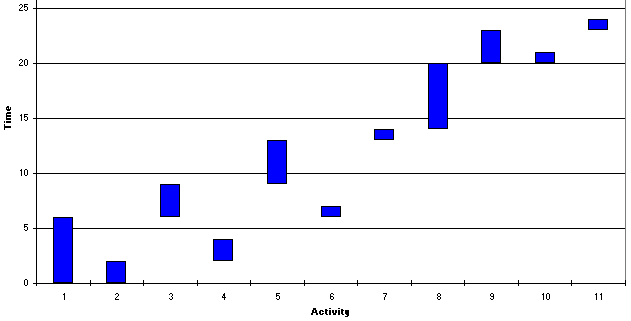Examining the resource profile the first point at which we now exceed the resource constraint of 8 is at time 20-21. Examining the Gantt chart this is associated with activity 9 (requiring 6 resource units) being performed simultaneously with activity 10 (which requires 6 resource units). Plainly one (or other) of these activities needs to be delayed. Here, we choose to delay the activity with the smallest completion time (activity 10) until activity 9 has finished. Hence we have a suggested start time for activity 10 of 23. Entering this into the spreadsheet we get: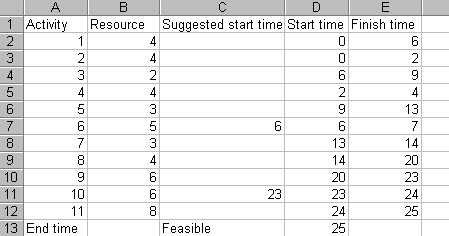which we can see is declared to be feasible (cell C13 above, i.e. it satisfies the resource constraint), with a resource profile of: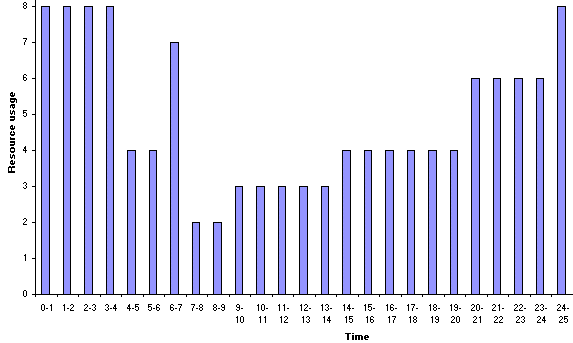Hence we have a feasible solution to the problem of determining the start time for each activity so as to respect the resource constraints and the network precedence relationships. This solution involves completing the project in 25 weeks. This may, or may not, be the optimal (minimum) project completion time when we have our resource constraint of 8 present.

Hence we can suggest a general procedure (algorithm) for estimating the minimum possible project completion time in the presence of resource constraints as follow:

• take the start times associated with the resource unconstrained project

Then repeat the following until the resource constraints are satisfied:

• examine the resource profile
• take the first point at which a resource constraint is violated and examine the activities associated with that violation. This violation must be associated with one (or more) activities starting.
• from the activities which were starting choose the activity with the smallest completion time
• attempt to remove the violation by preventing the chosen activity from starting until the next time at which an activity ends

Although we did not phrase our approach above in these terms you should be able to see that if we had set out this general approach first, and then applied it to the above example, we would have ended up with exactly the same solution.